# What Is A Biploar Junction Transistor (BJT) ? Explain the common base, common emitter and common collector configuration .

## What Is A Bipolar Junction Transistor ( BJT )

In our previous Diode tutorial , we learnt about the simple pn junction diodes which are made up of two pieces of semiconductor material, either silicon or germanium to form a  PN-junction .

If  two such single diodes are connected back to back , this will give us two pn-junctions connected together in series that share a common p or n terminal.

The fusion of  two single pn junction diodes produces a three layer, two junction, three terminal device known as Bipolar Junction Transistor, or BJT .

In other words, a bipolar transistor is a semiconductor device , consists of two pn junctions formed by sandwiching either p-type or n-type semiconductor between a pair of opposite types.

Accordingly, there are two types of bipolar transistors, such as :

1. n-p-n transistor
2. p-n-p transistor

In an n-p-n transistor , two n-type semiconductors are separated by a thin layer of p-type as shown in the figure below.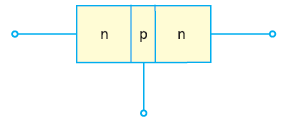Similarly, in a p-n-p transistor, two p-type semiconductors are separated by a thin layer of n-type as shown in figure below.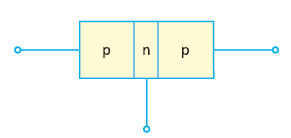As shown in both the figures , in each type of transistor, there are two pn junctions. Therefore, it may be regarded as a combination of two diodes that are connected back to back.

It has three terminals, one taken from each type of semiconductor.

The middle section of the transistor is a very thin layer. This plays an important role in the function of a transistor.

### Transistor Symbols & Terminals

A transistor whether n-p-n or p–n-p , has three section of doped semiconductors.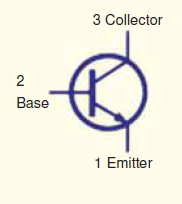The section on one side is called emitter and the section on the other side is knows as collector. The middle section is called the base and it  forms two junctions between the emitter and collector.

#### Emitter

The section on one side which supplies charge carriers (electrons or holes) is called as emitter. It is always forward biased with respect to the base in order to supply a large number of majority carriers.

In the figure below, the emitter (n-type) of the n-p-n transistor is forward biased and supplies electrons to its junction with the base.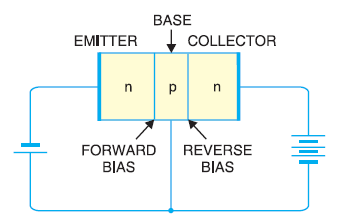Similarly, in the figure below, the emitter (p-type) of the p-n-p transistor is forward biased and supplies holes to its junction with the base.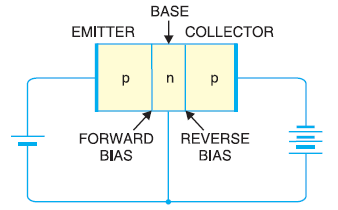#### Collector

The section on the other side which collects the charge carriers is known as collector.

The collector is always reverse biased with respect to base so that it can remove charges from its junction with the base.

The collector (n-type) of the n-p-n transistor has reverse bias and receives electrons. Similarly, the collector (p-type) of the p-n-p transistor has reverse bias and receives holes .

#### Base

The middle section which forms two pn junctions between  the emitter and collector is called as base.

The base-emitter junction is forward biased, thus allows a low resistance path for the emitter circuit.Whereas, the base-collector junction is reverse biased and provides a high resistance path in the collector circuit.

### Some Important Facts about Transistors

In a transistor, the base is much thinner than the emitter, however, the collector is wider than both base and emitter.

The emitter is heavily doped so that a large number of charge carriers become available to be injected into the base. The base is lightly doped so that it can pass most of the charge carriers that arrives from the base to the collector. The collector is moderately doped.

The emitter-base junction is always forward biased where as the base-collector junction is always reverse biased.

Since the resistance of the  emitter-base junction is very small as compared to the base-collector junction resistance, therefore the forward bias applied to the emitter-base junction is very small as compare to the reverse bias applied to the base-collector junction

## NPN Transistor Working

The figure below shows an npn transistor with its emitter-base junction forward biased by voltage VEB and base-collector junction reverse biased by voltage VCB.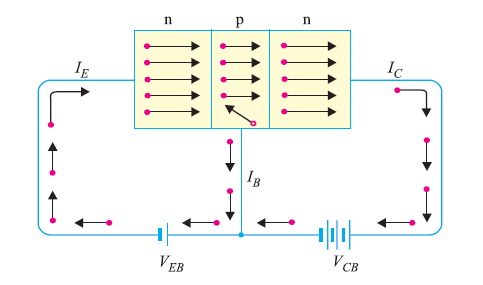The forward bias causes the electrons in the n-type emitter to flow towards the base. This constitutes the emitter current I.

Now as these electrons from emitter flow through the p-type base, they combine with the holes. As we already know, the base is lightly doped and very thin, hence, a very small amount of holes are available to be combined with the electrons (nearly 5%) from emitter  and constitute the base current IB.

The remaining electrons (more than 95%) cross over the base into the collector region and constitute collector current IC.

Now it is clear that the emitter current is the sum of base current and collector current.

IE = I + IC

## PNP Transistor Working

The figure below, shows a pnp transistor with emitter-base junction forward biased by voltage VEB  and base-collector junction reverse biased by voltage VCB.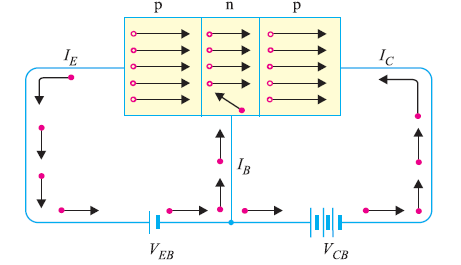The forward bias causes the holes in the p-type emitter to flow towards the base, thus constituting the emitter current IE.

As the holes cross the emitter and enter into the n-type base, they tend to combine with the electrons. Since the base is lightly doped and very thin , hence only a few holes (less than 5%) combine with the electrons and constitute base current IB.

The remainder holes (more than 95%) cross the base into the collector region and constitute collector current IC.

### Bipolar Transistor Configuration

A bipolar transistor has three terminals such as  emitter, base and collector. When a transistor is to be connected in an electronics circuit, we require four terminals; two for input and two for the output.

This problem is solved by making one terminal of the transistor common to both input and output terminals.

Hence, the input is applied between the common terminal and one of the other two terminals and the output is taken between the common terminal and the remaining terminal.

Accordingly, a transistor can be connected in a circuit in the following three ways :

1. Common base connection
2. Common emitter connection
3. Common collector connection

Each method of connection respond differently to its input signal within a circuit as the static characteristics of the transistor vary with each circuit arrangement.

#### Common Base Connection

In the common base configuration, the input is applied between emitter and base and output is taken between base and collector.

As the name suggests, the base of the transistor is common to both input and output circuit.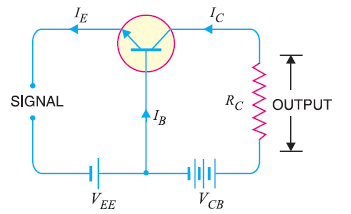The figure above shows a common base npn transistor circuit . The figure below shows a common base pnp transistor circuit.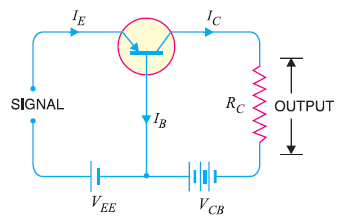#### Current Amplification Factor (α)

The current amplification factor is the ratio of output current to  the input current.

In a common base configuration, emitter current Iis the input current and collector current Iis the output current.

Hence, the ratio of change in collector current to the change in emitter current at constant collector base voltage VCB is known as current amplification factor.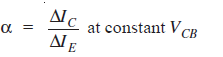Since the input current flowing into the emitter  is quite large as it is the sum of both the base current and collector current. Therefore, the collector current output is less than the emitter current input resulting in a current gain for this type of circuit of “1” (unity) or less.

Practical value of α range from 0.9 to 0.99.

#### Expression for Collector Current

The total collector current consists of :

1. part of emitter current which reaches the collector terminal i.e. αIE
2. Leakage current Ileakage , due to the movement of minority carriers across the base-collector junction as it is reverse biased. This is much smaller than  αIE.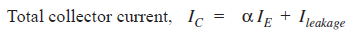When the emitter is open, i.e.  I= 0, a small leakage current sill flows in the collector circuit.

This Ileakage is abbreviated as ICBO , i.e. collector-base current with emitter open.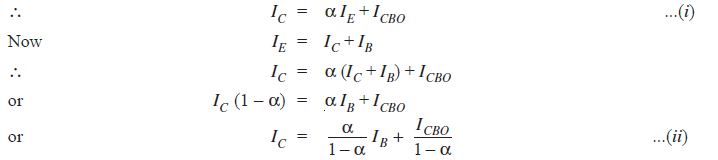Relation (i) or (ii) can be used to find IC .

Hence, It is clear from these relations that the collector current of a transistor can be controlled by either the emitter or base current.

#### Characteristics of Common Base Connection

The most important characteristics of common base connection are input characteristics and output characteristics.

#### Input Characteristics

It is the curve between emitter current Iand emitter-base voltage VBE at constant collector-base voltage VCB .

The VEB is taken along x-axis and I is taken along y-axis. The figure below shows the input characteristics of a typical transistor in Common base configuration.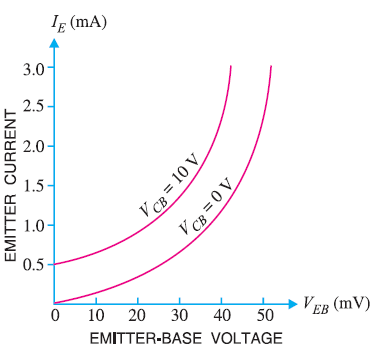The following points may be noted from there characteristics :

1. The emitter current IE increases rapidly with small increase in emitter-base voltage VEB which means that the input resistance is very small.
2. The emitter current is almost independent of collector-base voltage VCB . Therefore, the emitter current and hence the collector current is almost  independent of collector voltage

The ratio of change in emitter-base voltage to the resulting change in emitter current at constant collector-base voltage is known as input resistance. Hence,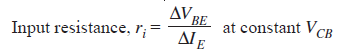The input resistance is quite small, of the order of a few ohms.

#### Output Characteristics

It is the curve between collector current Iand collector-base voltage VCB at constant emitter current IE.

The collector-base voltage is taken along the x-axis and collector current is taken along the y-axis.

The figure below shows the output characteristics of a typical transistor in CB arrangement.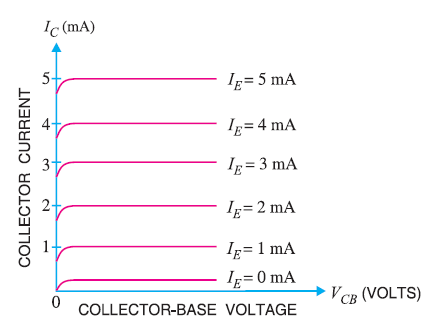The following points may be noted from the characteristics :

1. The collector current IC varies with   only at very low voltages (< 1V) .The transistor is never operated in this region.
2. When the value of VCB is increased above 1 -2 V, the collector current becomes constant as shown in fig.11 by the horizontal curves. It means that now Iis independent of  VCB and depends upon Ionly. The transistor is always operated in this region.
3. A very large change in collector-base voltage produces only a tiny change in collector current. This means that output resistance is very high.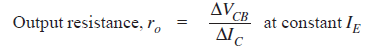The output resistance is very high of the order of several kilo-ohms.

#### Common Emitter Connection

In this circuit arrangement, input is applied between base and emitter and output is taken between collector and emitter. As the name suggests, here the emitter is common to both input and output circuits.

The figure below shows the common emitter npn transistor circuit.The figure below  shows the common emitter pnp transistor circuit.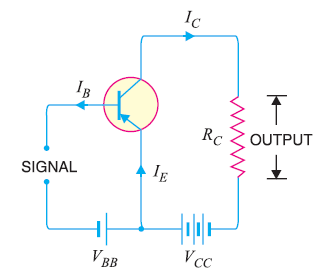#### Base Current Amplification factor (β)

The ratio of change in collector current to the change in base current is known as base current amplification factor(β).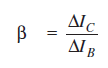Since, less than 5% of the emitter current flows as the base current, hence the value of β is generally greater than 20. Usually its value ranges between 20 to 500.

This type of connection is frequently used, as it gives appreciable current gain as well as voltage gain.

#### Relation between α and β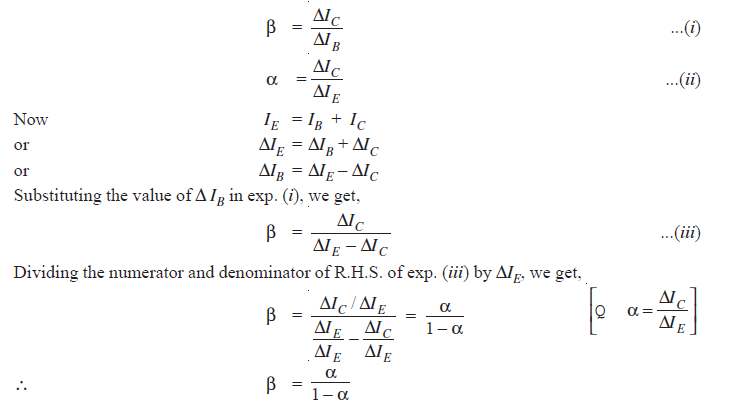It is clear from the above equation that when α approaches unity, β approaches infinity. In other words, the current gain in common emitter connection is very high. Hence this circuit arrangement is used in almost all transistor applications.

#### Expression for Collector Current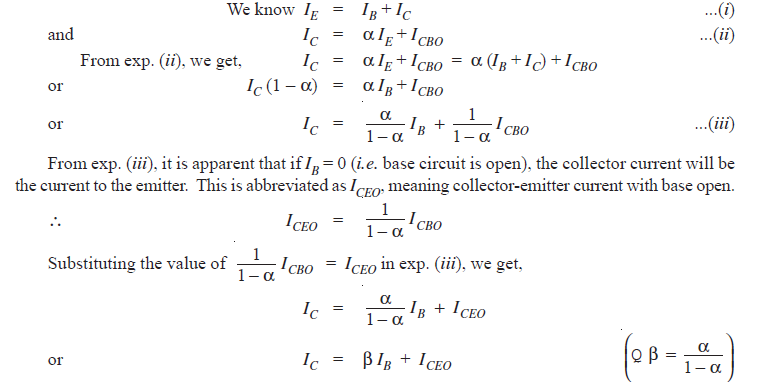#### Input Characteristics

It is the curve between the base current Iand base-emitter voltage VBE at constant collector-emitter voltage VCE .

The input characteristics of  a CE connection is shown in the figure below.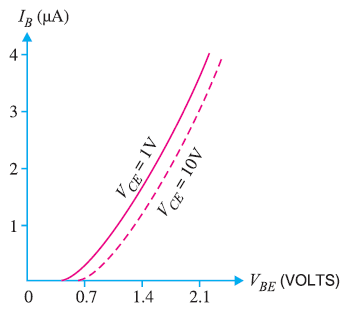Here the base-emitter voltage VBE is taken along the x-axis and base current Iis taken along the y-axis.

The following points may be noted from the characteristics :

1. The characteristics resembles that of a forward biased diode curve.
2. As compared to CB arrangement, Iincreases less rapidly with VBE. Therefore, input resistance of a CE circuit is higher than that of CB circuit.

The ratio of change in base-emitter voltage to the change in base current at constant VCE is known as input resistance.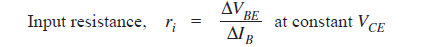The value of input resistance for a CE circuit is of the order of a few hundred ohms.

#### Output Characteristics

It is the curve between collector current Iand collector-emitter voltage VCE at constant base current I.

The output characteristics for a CE circuit is shown in the figure below.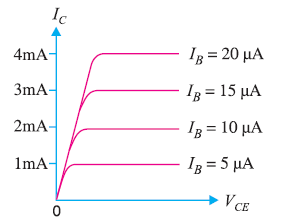Here  VCE is taken along the x-axis and Iis taken along the y-axis.

The following points may be noted from the characteristics :

1. The collector current Ivaries with VCE for VCE between 0 and 1 V only. After this, collector current becomes almost constant and independent of VCE. This value of VCE upto which collector current changes with VCE is called the knee voltage.
2. The transistors are always operated in the region above knee voltage.
3. Above knee voltage, Iis almost constant.
4. For any value of  VCE above knee voltage, the collector current Iis approximately equal to βIB.

The ratio of change in collector emitter voltage to the change in collector current at constant base current is known as the output resistance.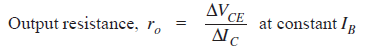The output resistance of a CE circuit is of the order of 50 K ohms.

#### Common Collector Connection

In this circuit configuration, input is applied between the base and collector and the output is taken between the emitter and collector terminals.

As the name suggests, collector of the transistor is common to both input and output circuits.

The figure below shows a common collector npn transistor circuit.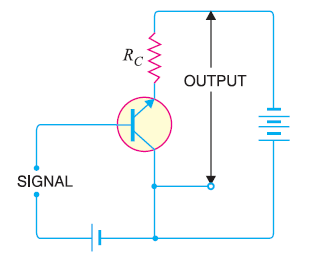The figure below shows common collector pnp circuit.#### Current Amplification Factor (γ)

The ratio of change in emitter current to the change in base current is knows as current amplification factor (γ) in common collector (CC) configuration.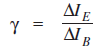This circuit provides about the same current gain as the common emitter circuit as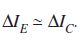However, its voltage gain is always less than 1.

#### Expression for Collector Current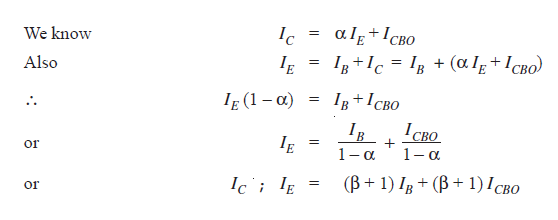The common collector configuration has very high input resistance and very low output resistance. Due to this reason, the voltage gain provided by this circuit is always less than 1. Therefore, CC circuit arrangement is never used for amplification. However, due to relatively high input resistance and low output resistance, this circuit is used for impedance matching i.e. for driving a low impedance load from a high impedance source.